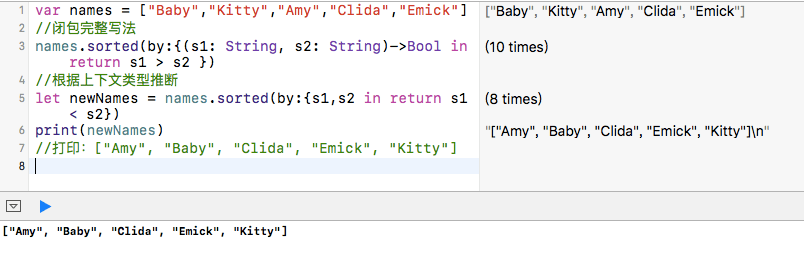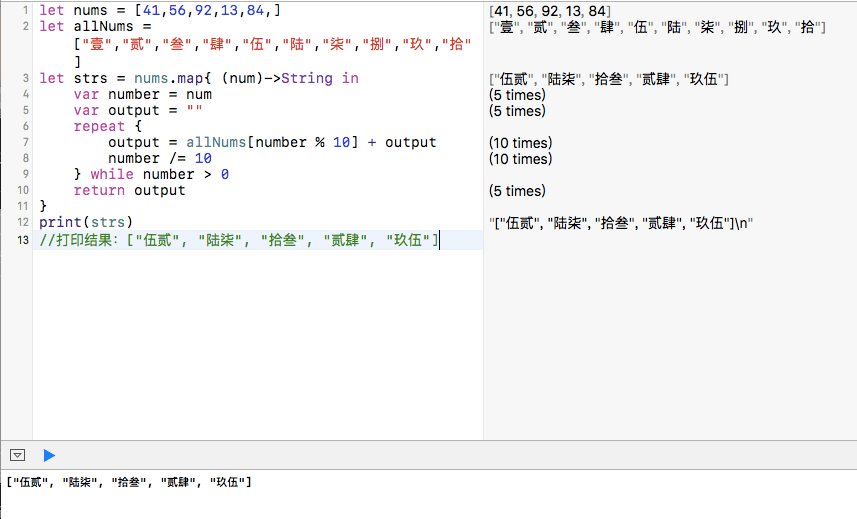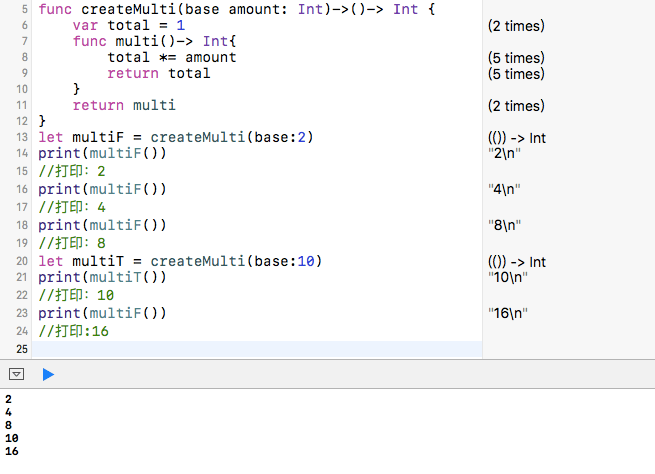# 闭包表达式

• 闭包表达式的语法大致形式如下：在大括号"{}"里面包含参数类型、返回值类型以及程序体。
``````{ ( parameters ) -> return type in
statements
}
``````

• 当闭包作为一个函数的参数传递过去的时候，这也是闭包最常使用的场景。比如对于数组排序，数组类型有个sorted(by:)函数，其接受一个(String, String)->Bool形式的闭包作为参数，这种情况下，Swift能够推断出闭包的参数和返回值类型，那么这些类型加上箭头(->）都可以省略不写。
``````var names = ["Baby","Kitty","Amy","Clida","Emick"]
//闭包完整写法
names.sorted(by:{(s1: String, s2: String)->Bool in return s1 > s2 })
//根据上下文类型推断
let newNames = names.sorted(by:{s1,s2 in return s1 < s2})
print(newNames)
//打印：["Amy", "Baby", "Clida", "Emick", "Kitty"]
``````• 如果闭包体里面只有一行表达式的话，return关键字也可以省略。
``````    names.sorted(by:{s1,s2 in s1 < s2})
``````
• Swift自动为内联闭包提供了实参名缩写功能，你可以直接通过\$0、\$1、\$2...来引用实参。如果你在闭包表达式中使用实参名缩写，你可以在定义中省略实参列表，并且缩写的实参名的个数和类型会通过函数类型进行推断。in关键词同样也可以被省略，因为此时闭包表达式完全由闭包主体构成。
``````    names.sorted(by:{ \$0>\$1 })
``````

# 后置闭包（trailing closure）

``````//假设有个这样的函数
func withAClosure(closure:()->void){
}
//一般函数调用，这样写
withAClosure(closure: {
//闭包体
}
)
//可以用后置闭包代替
withAClosure(){
//比包体
}
``````

``````//后置闭包写法
let newNames = names.sorted(){\$0 > \$1)
//括号可以省略了
let newNames1 = names.sorted{\$0 > \$1}
``````

``````let nums = [41,56,92,13,84,]
let allNums = ["壹","贰","叁","肆","伍","陆","柒","捌","玖","拾"]
let strs = nums.map{ (num)->String in
var number = num
var output = ""
repeat {
output = allNums[number % 10] + output
number /= 10
} while number > 0
return output
}
print(strs)
//打印结果：["伍贰", "陆柒", "拾叁", "贰肆", "玖伍"]
``````# 捕获值

Swift中最简单的闭包形式是嵌套函数。嵌套函数可以捕获外层函数所有的实参以及定义的常量和变量。

``````func createMulti(base amount: Int)->()-> Int {
var total = 1
func multi()-> Int{
total *= amount
}
return multi
}
let multiF = createMulti(base:2)
print(multiF())
//打印：2
print(multiF())
//打印：4
print(multiF())
//打印：8
let multiT = createMulti(base:10)
print(multiT())
//打印：10
print(multiF())
//打印:16
``````# 示例代码

https://github.com/99ios/23.9